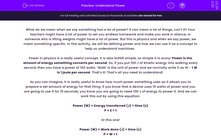# Understand Power

In this worksheet, students will learn the meaning of the term power and how to use it. They will also develop their knowledge of the power equation.Key stage:  KS 4

Year:  GCSE

GCSE Boards:   AQA, AQA Trilogy, OCR 21st Century, OCR Gateway, Pearson Edexcel, Eduqas, AQA Synergy,

Curriculum topic:   Energy, Explaining Motion, Forces, Energy: Forces Doing the Work, Movement and Interactions

Popular topics:   Physics worksheets

Difficulty level:#### Worksheet Overview

What do we mean when we say something has a lot of power? It can mean a lot of things, can't it? Your teachers might have a lot of power to set you endless homework and make you work in silence, or someone who is lifting weights might have a lot of power. But this is physics and when we say power, we mean something specific. In this activity, we will be defining power and how we can use it as a concept to help us understand machines.

Power in physics is a really useful concept. It is also SUPER simple, so simple it is scary! Power is the amount of energy something converts per second. So, if you put 100 J of kinetic energy into walking every second, then you have a power of 100 watts. ‘Watt’ is the unit of power and we normally write it as W - 1 W is 1 joule per second. That’s it! That’s all you need to understand!

As you can imagine, it is really useful to know how much power something uses as it allows you to prepare a set amount of energy for that thing. If you know that a device uses 10 watts of power and you are going to use it for 10 seconds, you know you are going to need 100 J of energy to power it. And we can work this out by using this equation:

Power (W) = Energy transferred (J) ÷ time (s)

P = E ÷ t

Or this one!

Power (W) = Work done (J) ÷ time (s)

P = W ÷ t

So, let’s have a go at an example:

Question:  A device uses 15 J of energy over 20 s. Calculate the power.

Step 1   Highlight all of the numbers in the equation:

A device uses 15 J of energy over 20 s. Calculate the power.

Step 2   Write out the numbers next to their symbols:

P = ?
E = 15 J
t = 20 s

Step 3   Put the numbers into the equation:

P = E ÷ t
P = 15 ÷ 20

P  = 0.75 W